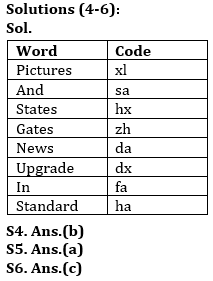Latest Banking jobs   »

# Reasoning Quiz For RBI Grade B Phase 1 2023-21st May

Directions (1-3): Study the following information carefully and answer the questions given below:
There are six poles in a ground. All poles are different height. Pole T is taller than U. Q is taller than S but shorter R. P is taller than U and Q which is just shorter than T. The third shortest pole is 40m. Pole R is not the tallest. Pole U is taller than S. Height of tallest pole is 80m. Height of pole T is a prime number. T is not taller than R.

Q1. Which of the following pole is tallest?
(a) U
(b) Q
(c) P
(d) T
(e) None of these

Q2. Which of the following may be the height of pole T?
(a) 63m
(b) 32m
(c) 55m
(d) 67m
(e) None of these

Q3. Which of the following pole is second shortest?
(a) T
(b) U
(c) S
(d) R
(e) None of these

Directions (4-6): Study the following information carefully and answer the questions given below:
In a certain code language:
‘States and gates pictures’ is written as ‘xl sa zh hx’,
‘News standard in upgrade’ is written as ‘ha fa dx da’,
‘Pictures upgrade and News’ is written as ‘sa dx xl da’ and
‘States pictures in News’ is written as ‘xl fa hx da’.

Q4. What is the code for ‘pictures upgrade’ in the given code language?
(a) xl fa
(b) xl dx
(c) da fa
(d) dx da
(e) None of these

Q5. What is the code for ‘standard’ in the given code language?
(a) ha
(b) fa
(c) dx
(d) da
(e) None of these

Q6. What is the code for ‘upgrade’ in the given code language?
(a) ha
(b) fa
(c) dx
(d) da
(e) None of these

Q7. In the given number ‘567823416’ if ‘2’ is added to each odd digit and ‘1’ is subtracted from each even digit, then how many digits are not repeated in the resultant thus obtained?
(a) One
(b) Two
(c) Three
(d) Four
(e) None

Q8. If each vowel of the word “RESPONSIBLE” is changed to its next in the English alphabetical series and each consonant to its previous letter in the English alphabetical series and then the alphabets thus formed are arranged in alphabetical order from left to right, which of the following will be seventh from the right end?
(a) K
(b) J
(c) M
(d) O
(e) None of these

Q9. Veer is 28th from the left end of a row and Aman is 22th from the right end of row. If they interchanged their positions then Aman ranks become 13th from right end. Find total number of persons in the row?
(a) 40
(b) 41
(c) 38
(d) 39
(e) None of these

Q10. Anurag ranked 42nd from the bottom and 6th from the top among those who passed an exam. Seven boys did not participate in the exam and three failed in it. How many boys were there in the class?
(a) 47
(b) 48
(c) 52
(d) 68
(e) 57

Solutions

Solutions (1-3):
Sol. P (80) > R > T > Q (40) > U > S

S1. Ans.(c)
S2. Ans.(d)
S3. Ans.(b)S7. Ans.(b)
Sol. ‘567823416’ changes to ‘759715335’ two numbers i.e., 9 and 1 are not repeated.

S8. Ans(a)
Sol.
Given word- RESPONSIBLE
Obtained word- AFFJKMOPQRR

S9. Ans(a)
Sol.
Total number of persons in the row = (28+13-1) = 40

S10. Ans(e)
Sol.
Number of boys who passed = (42 + 6 – 1) = 47
∴ Total number of boys in the class = (47 + 7 + 3) = 57## FAQs

### How many sections are there in the RBI Grade B Phase 1 Exam?

There are 4 sections in the RBI Grade B Phase 1 Exam i.e. English Language, General Awareness, Quantitative Aptitude & Reasoning.

#### Congratulations!Union Budget 2023-24: Free PDF